# Mock exam paper A Higher Level

Pre- Junior Certificate Examination, 2011

Technical Graphics

Higher Level

Section A

(120 marks)

Instructions

(a) Answer any ten questions in the spaces provided.

All questions carry equal marks.

(b) Construction lines must be clearly shown.

(c) All measurements are in millimetres.

(d) This booklet must be handed up at the end of the

examination.

(e) Write your name in the box provided

above and on all other pages used.

Note: Section B starts on Page 7

 NAME: SCHOOL: CLASS: TEACHER

SECTION A. Answer any ten questions. All questions carry equal marks

1.

Fill in the label for each diagram by selecting from the following four options: CHORD / RADIUS / SECTOR / ARC.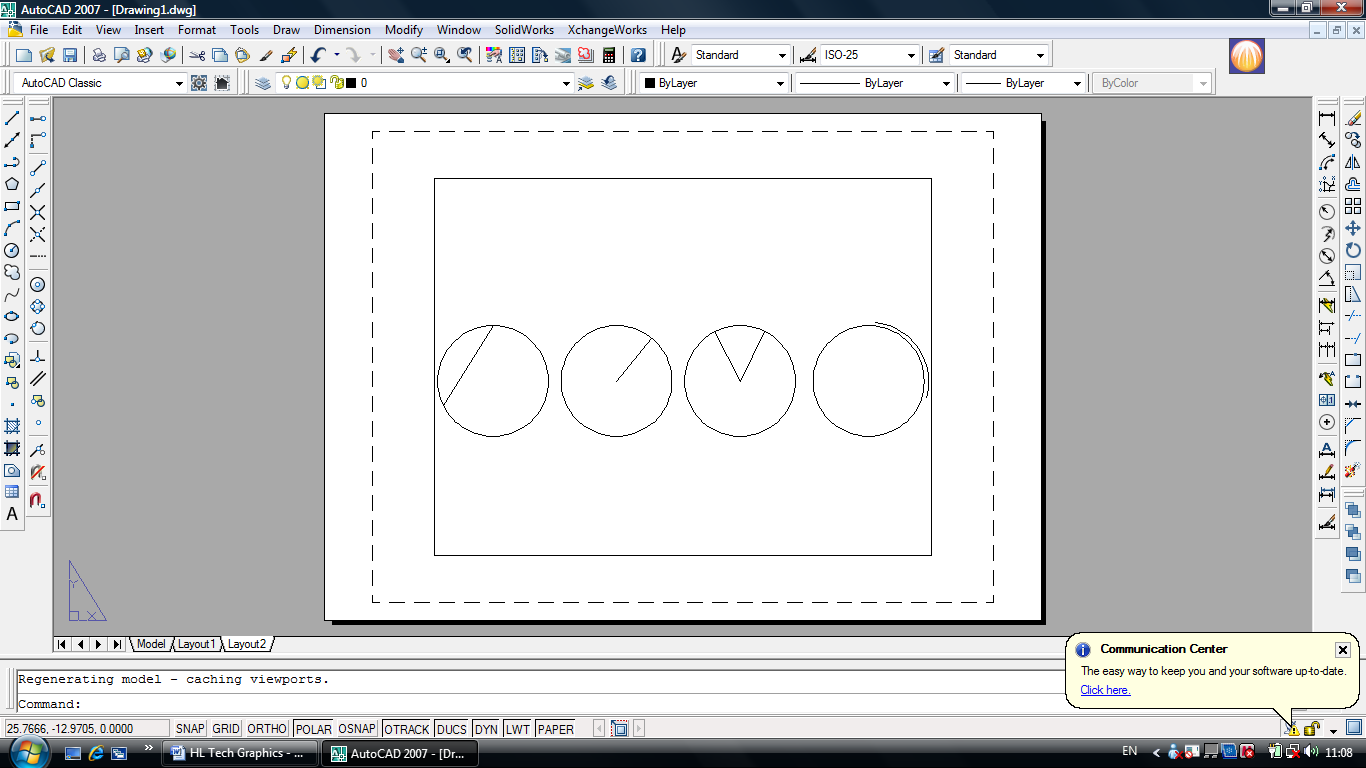2.

Below is the profile of a bicycle chain link. Show the constructions required to draw one of the arcs.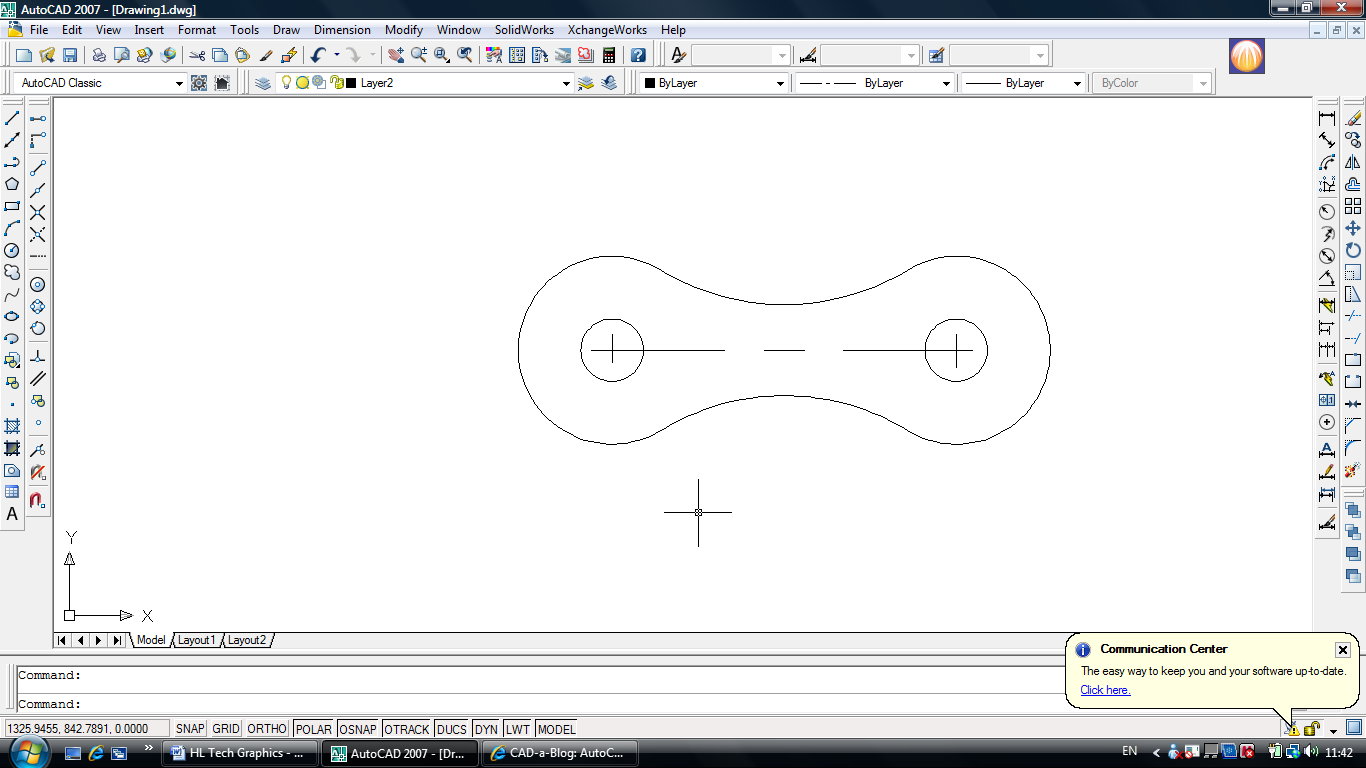3.

State the type and size of the angles shown.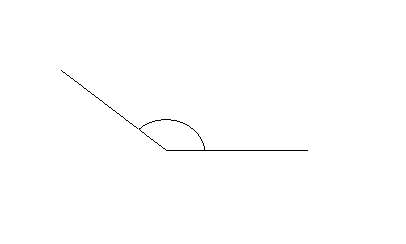Type: Size: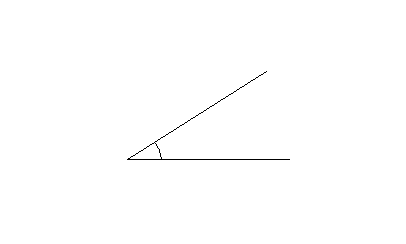Type: Size: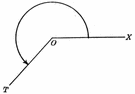Type: Size:

4.

 The Elevation, Plan & End View of a modern bench design are shown below. Complete the pictorial sketch of this bench in the grid provided.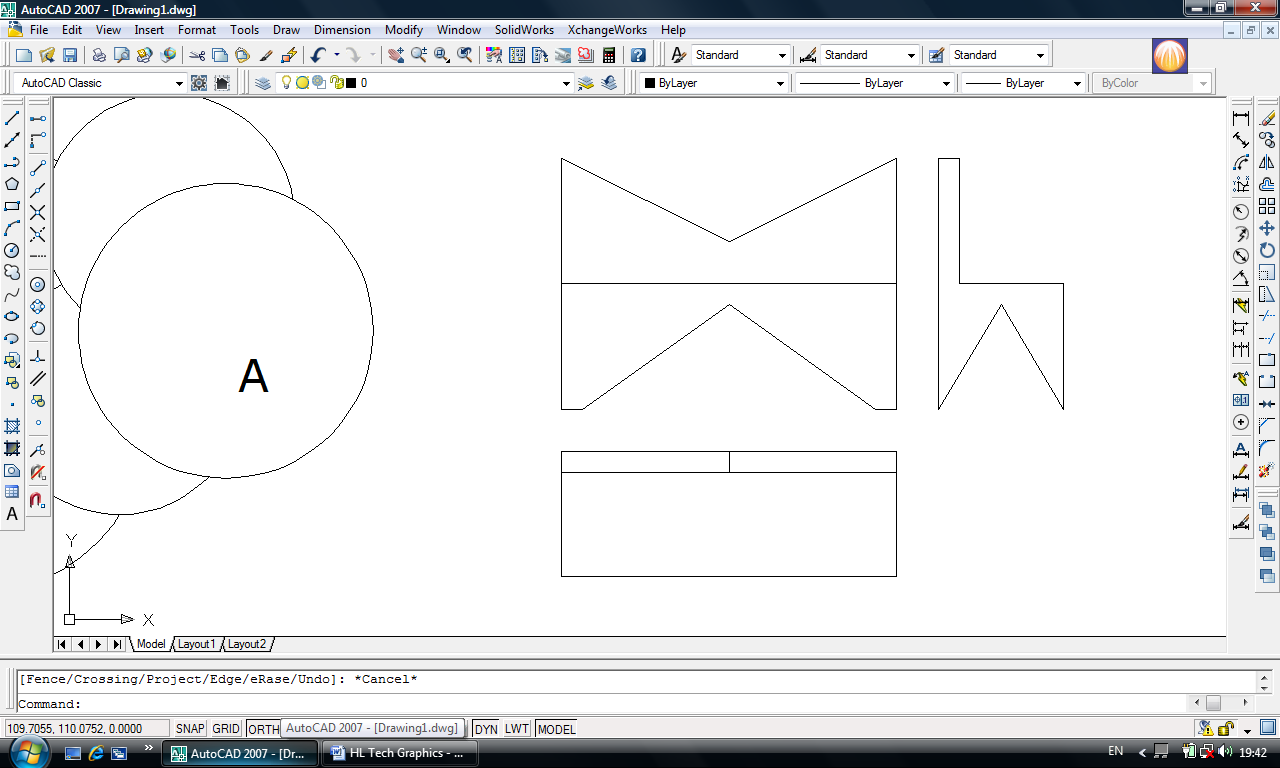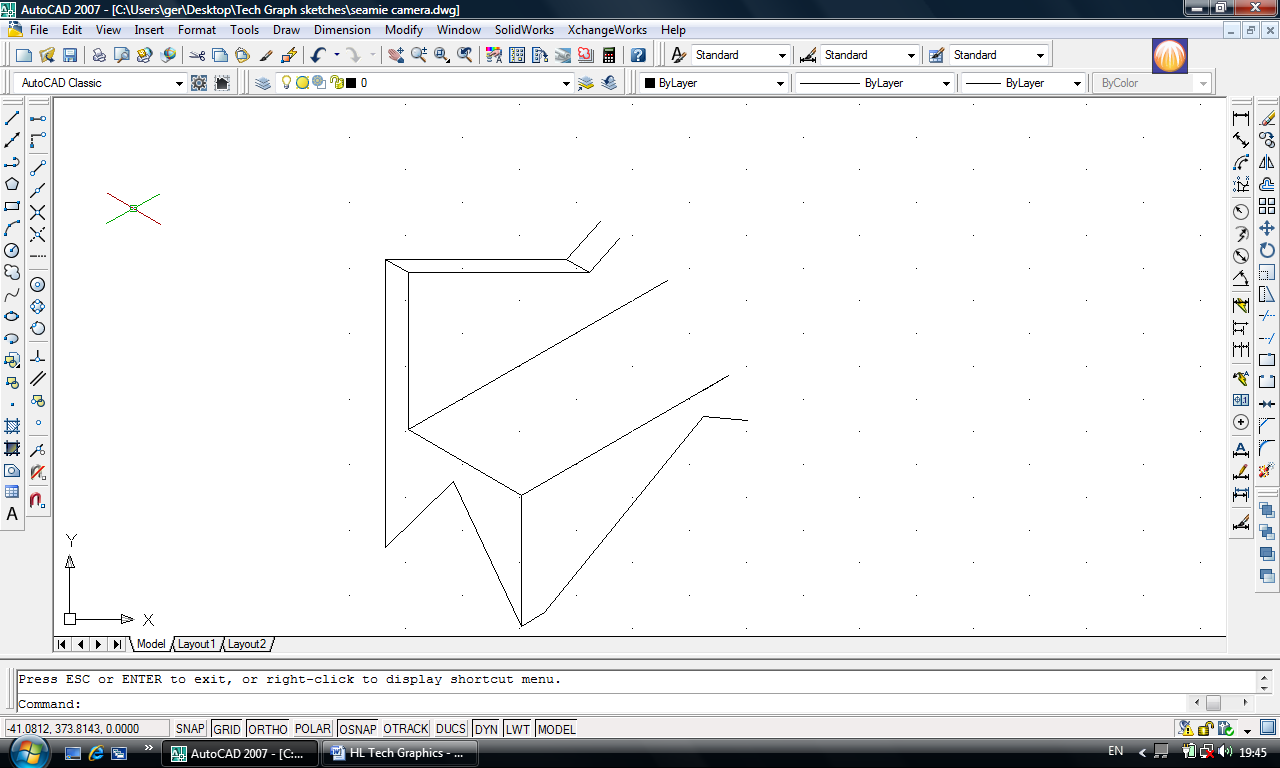5.

What do each of the following signs represent?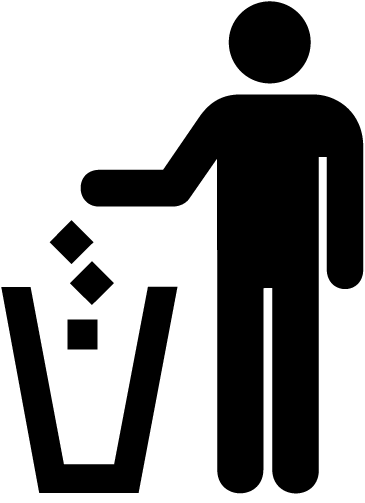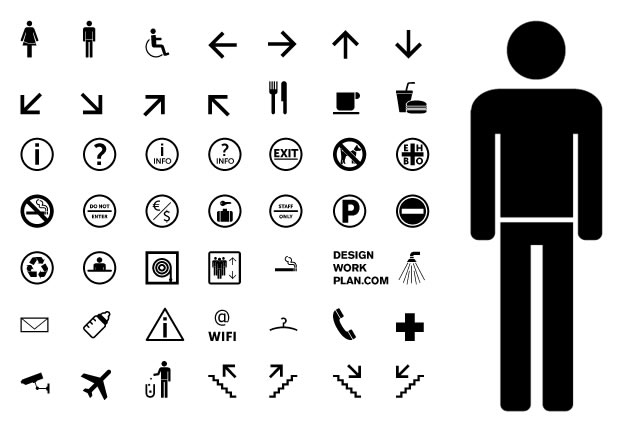Graphics which convey information without using language are known as_______________

6.

In the grid below label X, -X, Y, -Y. Draw the figure ABCD using the given co-ordinates.

 A (-20,30)B (50,30)C (30,-20)D (-60,-30)E (40,-10)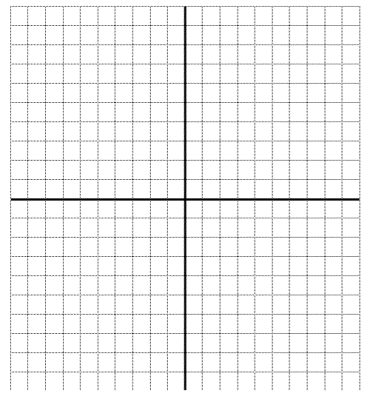7.The elevation and plan of a kettle are shown.In the space provided, draw a freehand pictorial sketch of the joystick.Colour or shade the sketch.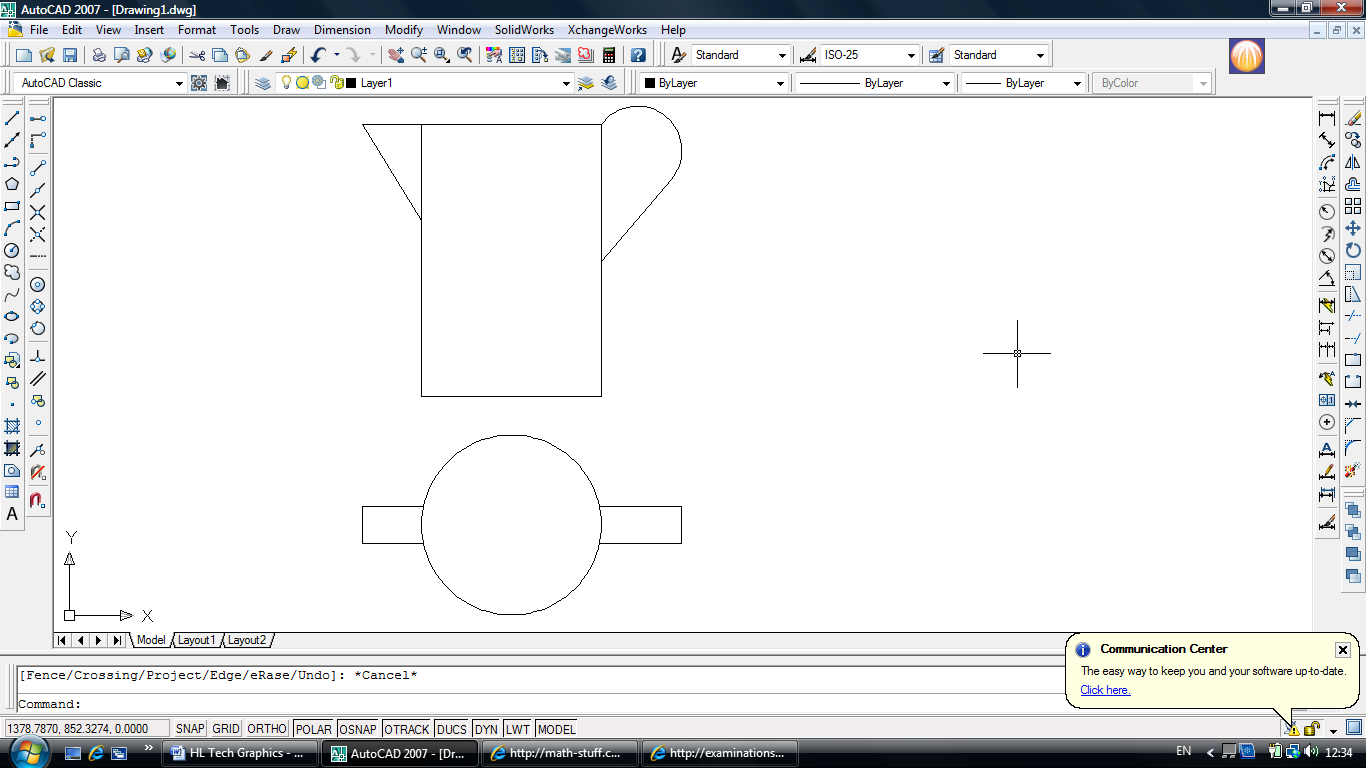8.

List the CAD commands used to edit the figure as shown in the sequence below.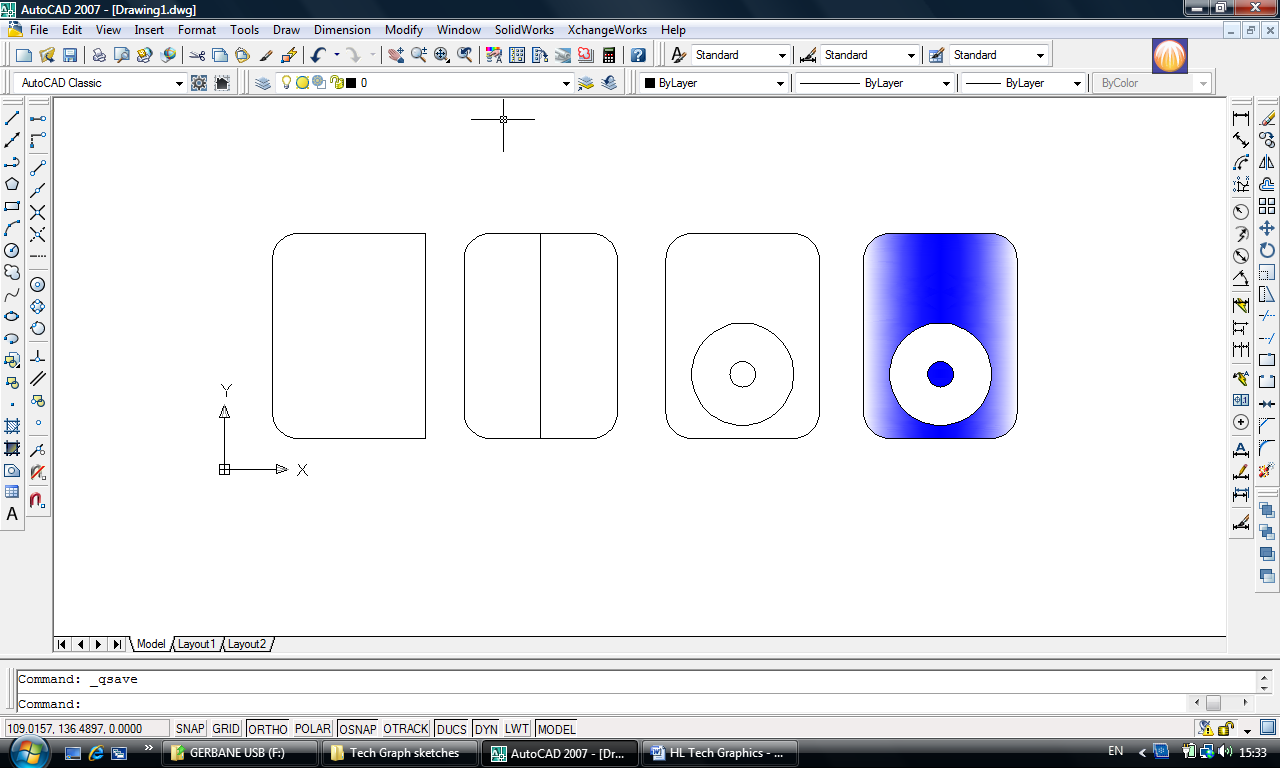________________ ________________ ________________ _______________

9.

The figure shows the letter P. Apply a central symmetry of the letter P bringing all relevant points through Y.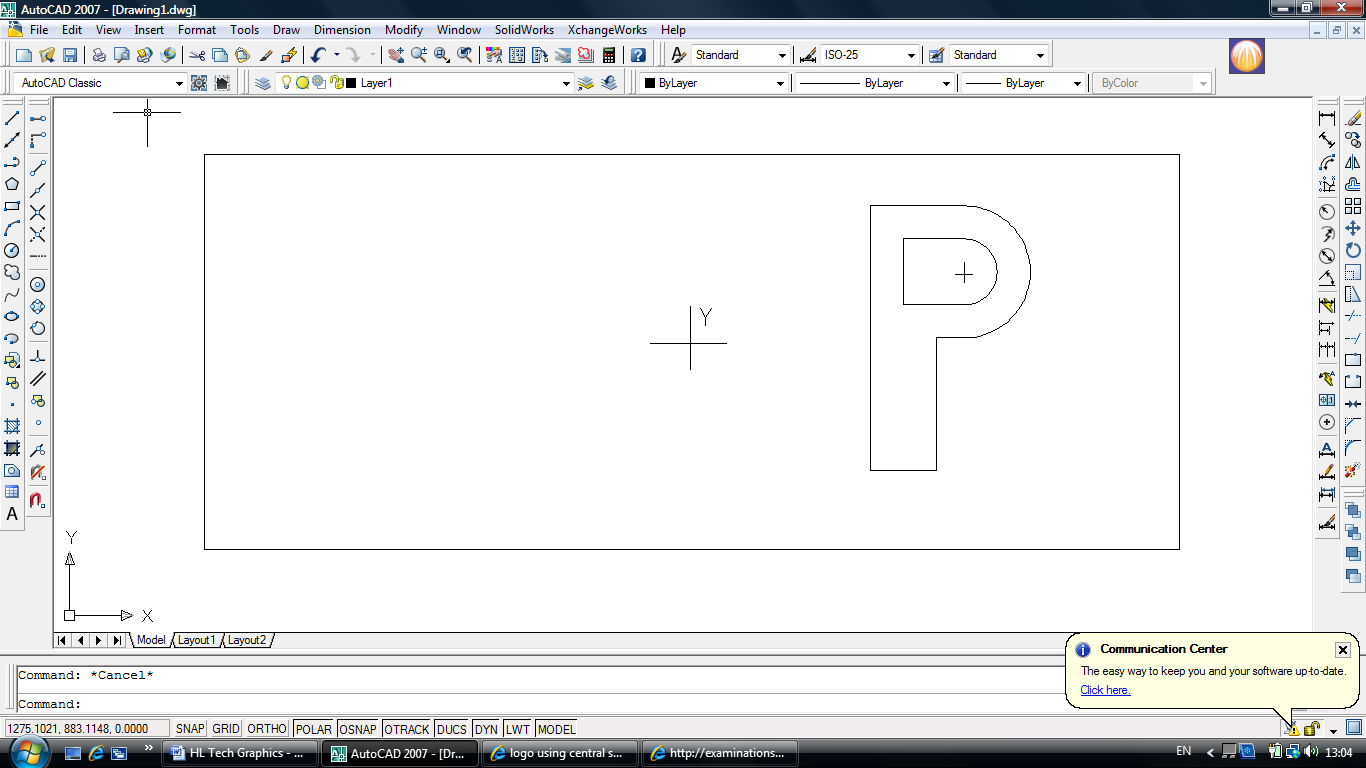10.

 The figure opposite shows a puzzle of 5 discs stacked loosely.Disc A is on top as shown. List the order in which they are stacked from the top down (A,B,C,D,E).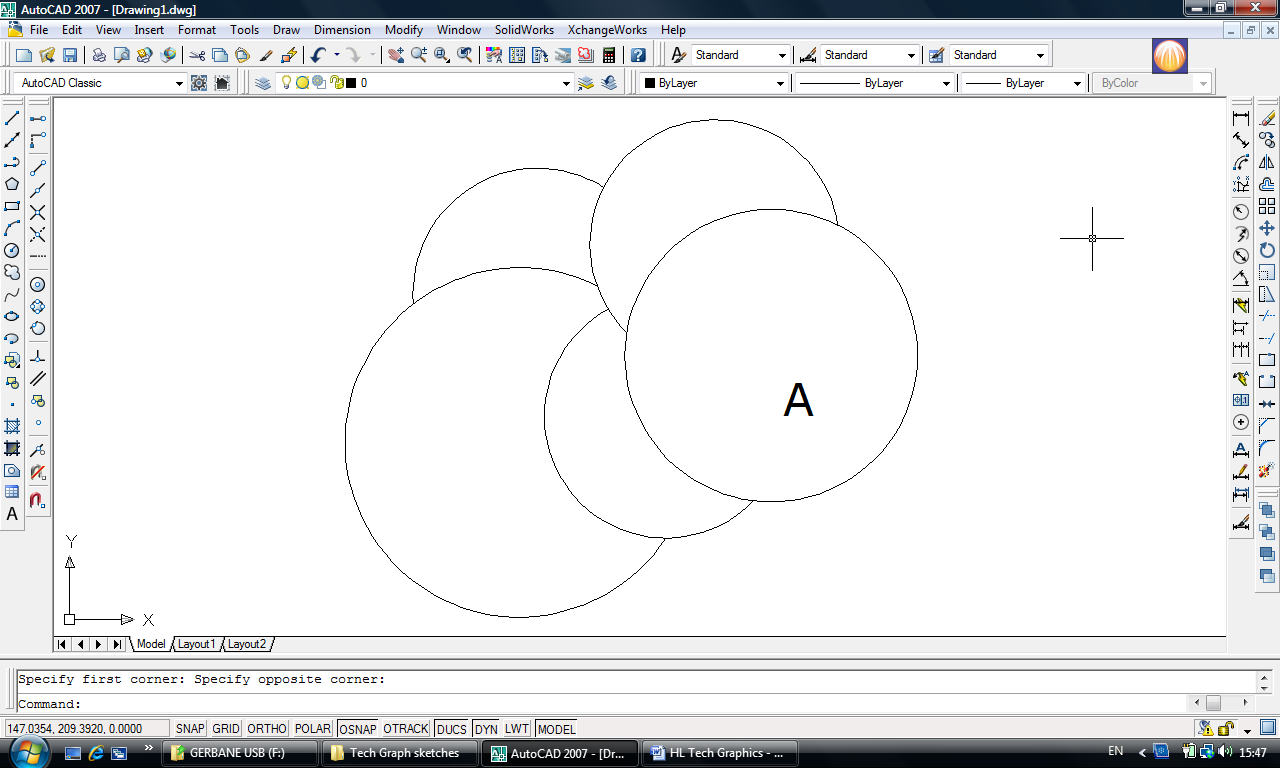1 A 2 3 4 5

11.

Starting at point L, show the perimeter of the given figur...